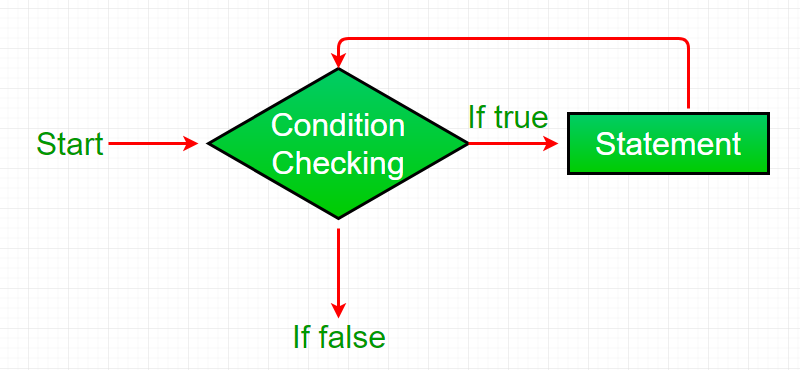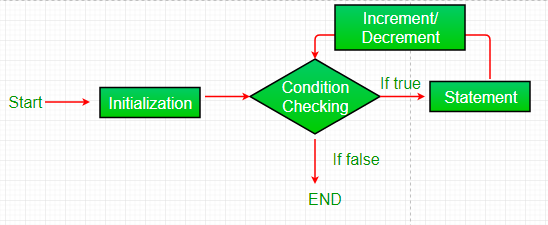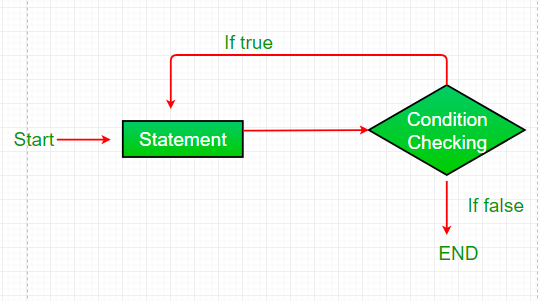# JavaScript For Loops

### 1.while loop:-

A while loop is a control flow statement that allows code to be executed repeatedly based on a given Boolean condition. The while loop can be thought of as a repeating if statement.
Syntax :

while ( boolean  condition);

{

loop   statements

}

Flowchart:-Example:-

`<script type = ``"text/javaScript"``>`
`// JavaScript program to illustrate while loop`
` `
`    ``var``x = 1;`
` `
`    ``// Exit when x becomes greater than 4`
`    ``while``(x <= 4)`
`    ``{`
`        ``document.write(``"Value of x:"``+ x + ``"<br />"``);`
` `
`        ``// increment the value of x for`
`        ``// next iteration`
`        ``x++;`
`    ``}`
` `
`< /script>`
Output:
value of x: 1
value of x: 2
value of x: 3
value of x: 4

### 2. For  loop:-

for loop provides a concise way of writing the loop structure. Unlike a while loop, a for statement consumes the initialization, condition and increment/decrement in one line thereby providing a shorter, easy to debug structure of looping.

Syntax:

for (initialization condition; testing condition;  increment/decrement)

{

statement(s)

}

Flowchart:-Example:-

`<script type = ``"text/javaScript"``>`
`// JavaScript program to illustrate for loop`
` `
`    ``var``x;`
` `
`    ``// for loop begins when x=2`
`    ``// and runs till x <=4`
`    ``for``(x = 2; x <= 4; x++) `
`    ``{`
`        ``document.write(``"Value of x:"``+ x + ``"<br />"``);`
`    ``}`
` `
`< /script>`
Output:-
value of x:2
value of x:3
value of x:4

### 3. do while loop:-

do while loop is similar to while loop with only difference that it checks for condition after executing the statements, and therefore is an example of Exit Control Loop.
Syntax:

do

{

statement

}   while (condition);

Flowchart:-Example:-

`<script type = ``"text/javaScript"``>`
`// JavaScript program to illustrate do-while loop`
` `
`    ``var` `x = 21;`
` `
`    ``do`
`    ``{`
`        ``// The line while be printer even`
`        ``// if the condition is false`
`        ``document.write(``"Value of x:"` `+ x + ``"<br />"``);`
` `
`        ``x++;`
`    ``} ``while` `(x < 20);`
` `
`< /script>`
Output:-
value of x:21